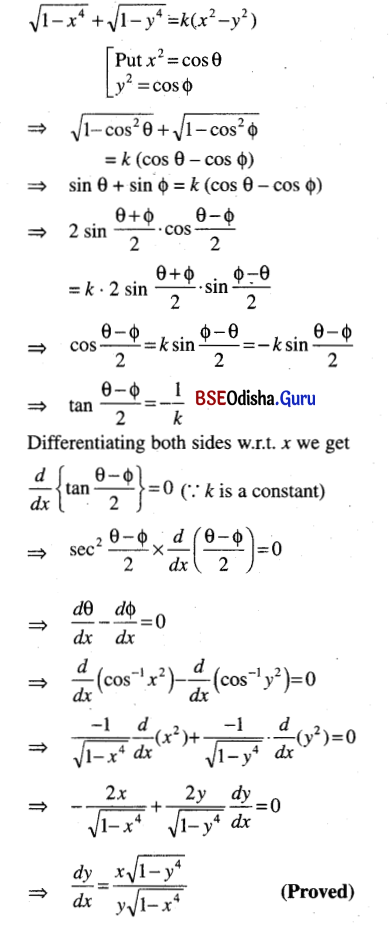# CHSE Odisha Class 12 Math Solutions Chapter 7 Continuity and Differentiability Ex 7(g)

Odisha State Board Elements of Mathematics Class 12 CHSE Odisha Solutions Chapter 7 Continuity and Differentiability Ex 7(g) Textbook Exercise Questions and Answers.

## CHSE Odisha Class 12 Math Solutions Chapter 7 Continuity and Differentiability Exercise 7(g)

Find $$\frac{d y}{d x}$$
Question 1.
xy2 + x2y + 1 = 0
Solution:
xy2 + x2y + 1 = 0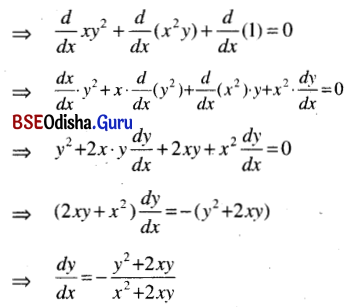Question 2.
$$x^{\frac{1}{2}} y^{-\frac{1}{2}}$$ + $$x^{\frac{3}{2}} y^{-\frac{3}{2}}$$ = 0
Solution: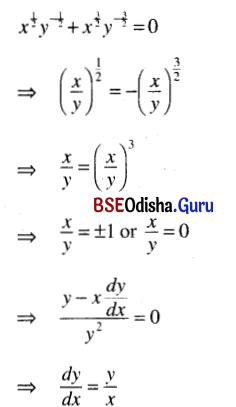Question 3.
x2 + 3y2 = 5
Solution:
x2 + 3y2 = 5
⇒ $$\frac{d}{d x}$$(x2) + 3 $$\frac{d}{d x}$$(y2) = 0
⇒ 2x + 6y$$\frac{d y}{d x}$$ = 0
⇒ $$\frac{d}{d x}$$ = –$$\frac{x}{3 y}$$

Question 4.
y2 cot x = x2 cot y.
Solution:
y2 cot x = x2 cot y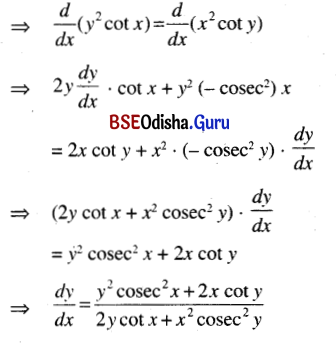Question 5.
y = tan xy
Solution:
y = tan xy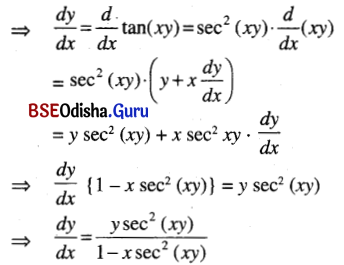Question 6.
x = y In (xy).
Solution:
x = y In (xy).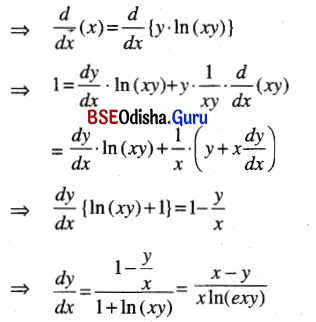Question 7.
exy + y sin x = 1
Solution:
exy + y sin x = 1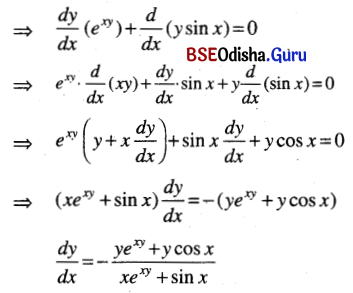Question 8.
In $$\sqrt{x^2+y^2}$$ = tan-1 $$\frac{y}{x}$$
Solution: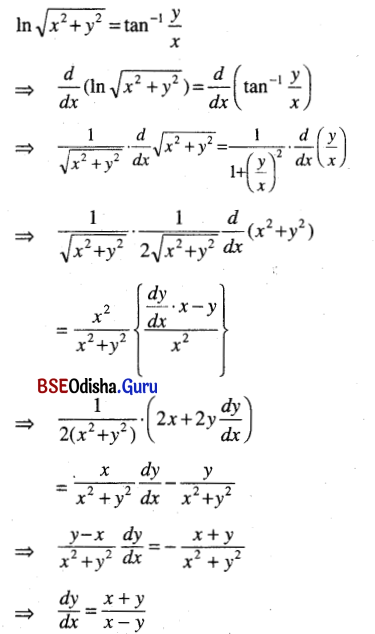Question 9.
yx = xsin y
Solution:
yx = xsin y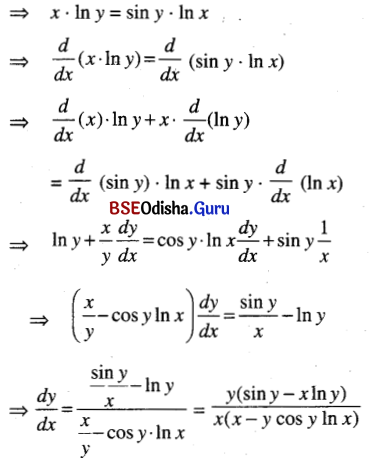Question 10.
If sin (x + y) = y cos (x + y) then prove that $$\frac{d y}{d x}$$ = –$$\frac{1+y^2}{y^2}$$.
Solution: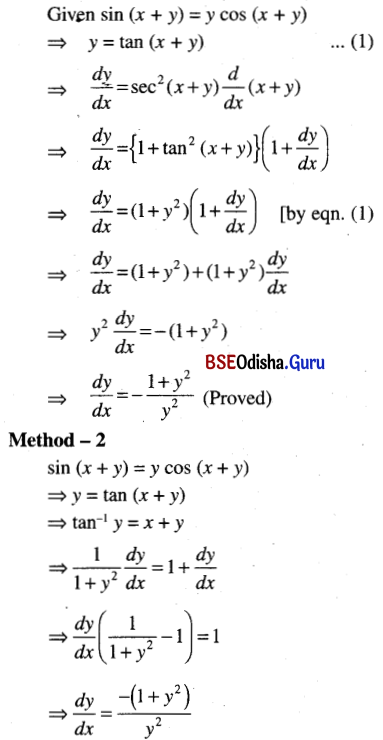Question 11.
If $$\sqrt{1-x^4}$$ + $$\sqrt{1-y^4}$$ = k(x2 – y2) then show that $$\frac{d y}{d x}$$ = $$\frac{x \sqrt{1-y^4}}{y \sqrt{1-x^4}}$$.
Solution: